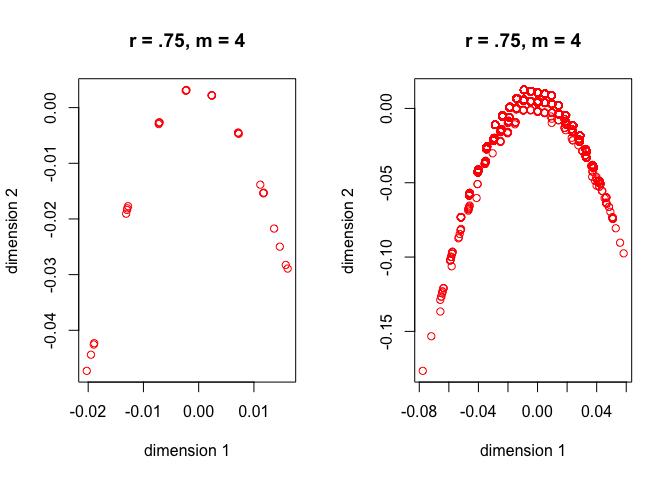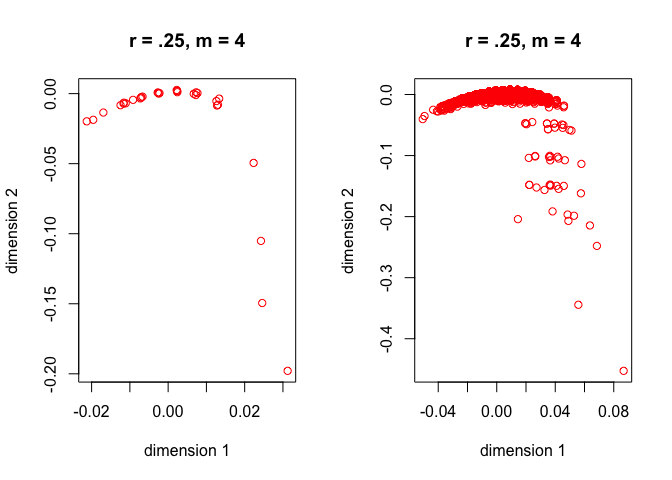croissants and wedges.

Note: This is a working paper which will be expanded/updated frequently. The directory deleeuwpdx.net/pubfolders/croissants has a pdf copy of this article, the complete Rmd file with all code chunks, and R and C files with the code. Unfortunately the Norway data cannot be shared, so that part of the paper is not reproducible. If you want to knit the Rmd file, remove the Norway section.

# 1 Introduction

In multiple correspondence analysis (MCA) we frequently observe two-dimensional scatter plots in which the points are on or close to a convex function, with shape similar to a parabola. This is often referred to as the horseshoe effect, but that name is not quite appropriate, since horseshoes fold back at the endpoints. Thus we suggest to call such plots croissants, which seems especially suitable if there is considerable dispersion around the convex function.

Croissants have a complicated history. In data analysis they were first described in the context of scale analysis by Guttman (1950). The MCA eigenvectors of perfect scales satisfy a second order difference equation, whose solution are the discrete orthogonal polynomials. The first and second component define the croissant. In the French data analysis literature the horseshoe or croissant is consequently called the Effet Guttman.

The next major contribution was Lancaster (1958), who described a family of bivariate distributions for which the singular value decomposition of the density consisted of orthogonal polynomials. The most famous member of the family is the bivariate normal, which has the Hermite-Chebyshev polynomials as its components. Lancaster’s work was generalized and extended by his students and co-workers. It was taken up by Benzécri’s school, in particular in the thesis of Naouri (1970).

In ecology horseshoes were first discussed in the influential paper of Hill (1974). They were considered a nuisance, and various techniues were developed to get rid of them (Hill and Gauch 1980). Ecologists work with the Gaussian abundance model for environmental gradients, and Ihm and van Groenewoud (1975) showed this resulted in horseshoes.

In multidimensional scaling quadratic structures were first discussed and analyzed by Levelt, Van De Geer, and Plomp (1966) for musical intervals (a parabola) and by De Gruijter (1967) for political parties (an ellipse). More information on horseshoes in the MDS context is in Diaconis, Goel, and Holmes (2008) and J. De Leeuw (2007).

The application of Hermite-Chebyshev polynomials to the multivariate normal case in MCA was outlined in section 3.8 of De Leeuw (1974). Gifi (1980) pointed out for the first time that oscillation matrices and total positivity were the relevant mathematical theories, see also section 9.2 of Gifi (1990). Total positivity was used in a general theory by Schriever (1983), Schriever (1985) that covers all previously discussed special cases. The appropriate way to patch the decomposition of the various bivariate marginals together in the multivariate case is due to De Leeuw (1982), see also Bekker and De Leeuw (1988). Much of the work of the Gifi school on horseshoes was summarized in Van Rijckevorsel (1987). #MCA

We give a very brief introduction to MCA to fix the terminology. We start with n measurements on m categorical variables. Variable j has $$k_j$$ categories. We then expand each variable to an indicator matrix $$G_j$$, indicating category membership. Thus $$G_j$$ is an $$n\times k_j$$ binary matrix with exactly one element equal to one in each column, and zeroes everywhere else. Define $$G:=\begin{bmatrix}G_1\mid\cdots\mid G_m\end{bmatrix}$$ and $$C=G'G$$. The matrix $$C$$, which contains the bivariate cross tables of the variables, is called the Burt Matrix (Tableau de Burt). Also define $$D:=\mathbf{diag}(C)$$, the diagonal matrix of univariate marginals. Both $$C$$ and $$D$$ are of order $$K:=\sum k_j$$.

MCA is defined as solving the generalized eigenvalue problem $$CY=mDY\Lambda$$, with $$Y'DY=I$$. The generalized eigenvectors $$Y$$ are called the category quantifications, and the centroids $$X:=\frac{1}{m}GY$$ are called the object scores. In most cases we do not use all eigenvectors in the data analysis but only a small number of them. The category quantifications are in an $$\sum k_j\times p$$ matrix, and the object scores are in an $$n\times p$$ matrix. Note that with the normalization we have chosen $$0\leq\Lambda\leq I$$ and $$X'X=\frac{1}{m}\Lambda$$.

In our computations we use the package geigen (Hasselman and Lapack authors 2015). Or, alternatively, we define $$E:=D^{-\frac12}CD^{-\frac12}$$, compute eigenvalues $$Z$$ such that $$EZ=mZ\Lambda$$, and then set $$Y=D^{-\frac12}Z$$.

Also, because of the singularities in $$G$$, there is one generalized eigenvalue equal to one (with a corresponding generalized eigenvector $$y$$ that has all elements equal) and there are $$m-1$$ generalized eigenvalues equal to zero.

# 2 Synthetic Data: Normal Distribution

One simple way to reliably generate croissants is to discreticize a sample from a multinormal. Of course there are various parameters to consider. The function discreteNormal() allows one to choose the size of the sample n, the number of variables m, the correlation between the variables r, and the discretization points (aka knots).

formals ("discreteNormal")
## $n ## ## ##$m
##
##
## $r ## ## ##$knots

Throughout this section we choose $$n=10,000$$. Our first normal croissant (category quantifications on the left, object scores on the right) has four variables, knots at the integers from -3 to +3, and all correlations equal to .75. We see somewhat more scatter or filling in the object score croissant, basically because object scores are convex combinations of category quantifications.Figure 1: Normal Croissants, m = 4, r = .75

If we decrease the correlation to .25 the croissant crumbles. A correlation of .25 is too close to independence, which means that not enough variation will be captured by the first two eigenvalues. Nevertheless if we discard the outliers the croissant is still there.Figure 2: Normal Croissants, m = 4, r = .25

In the third simulation we increase the number of variables to 25, with correlation .75. The croissants are tight and symmetric.